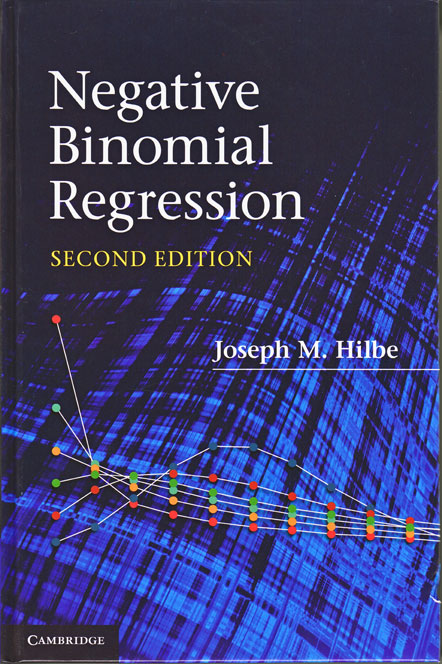# Negative Binomial Regression

Negative Binomial Regression, Second Edition, by Joseph M. Hilbe, reviews the negative binomial model and its variations. Negative binomial regression—a recently popular alternative to Poisson regression—is used to account for overdispersion, which is often encountered in many real-world applications with count responses.

Negative Binomial Regression covers the count response models, their estimation methods, and the algorithms used to fit these models. Hilbe details the problem of overdispersion and ways to handle it. The book emphasizes the application of negative binomial models to various research problems involving overdispersed count data. Much of the book is devoted to discussing model-selection techniques, the interpretation of results, regression diagnostics, and methods of assessing goodness of fit.

Hilbe uses Stata extensively throughout the book to display examples. He describes various extensions of the negative binomial model—those that handle excess zeros, censored and truncated data, panel and longitudinal data, and data from sample selection.

Negative Binomial Regression is aimed at those statisticians, econometricians, and practicing researchers analyzing count-response data. The book is written for a reader with a general background in maximum likelihood estimation and generalized linear models, but Hilbe includes enough mathematical details to satisfy the more theoretically minded reader.

This second edition includes added material on finite-mixture models; quantile-count models; bivariate negative binomial models; and various methods of handling endogeneity, including the generalized method of moments.

Preface to the Second Edition

1. INTRODUCTION

What is a negative binomial model?
A brief history of the negative binomial
Overview of the book

2. THE CONCEPT OF RISK

Risk and 2 × 2 tables
Risk and 2 × k tables
Risk ratio confidence intervals
Risk difference
The relationship of risk to odds ratios
Marginal probabilities: joint and conditional

3. OVERVIEW OF COUNT RESPONSE MODELS

Varieties of count response model
Estimation
Fit considerations

4. METHODS OF ESTIMATION

Derivation of the IRLS algorithm

Solving for ? L or U — the gradient
Solving for ?2 L
The IRLS fitting algorithm

Newton–Raphson algorithms

Derivation of the Newton–Raphson
GLM with OIM
Parameterizing from ? to x??
Maximum likelihood estimators

5. ASSESSMENT OF COUNT MODELS

Residuals for count response models
Model fit tests

Information criteria fit tests

Validation models

6. POISSON REGRESSION

Derivation of the Poisson model

Derivation of the Poisson from the binomial distribution
Derivation of the Poisson model

Synthetic Poisson models

Construction of synthetic models
Changing response and predictor values
Changing multivariable predictor values

Example: Poisson model

Coefficient parameterization
Incidence rate ratio parameterization

Predicted counts
Effects plots
Marginal effects, elasticities, and discrete change

Marginal effects for Poisson and negative binomial effects models
Discrete change for Poisson and negative binomial models

Parameterization as a rate model

Exposure in time and area
Synthetic Poisson with offset
Example

7. OVERDISPERSION

What is overdispersion?
Handling apparent overdispersion

Creation of a simulated base Poisson model
Delete a predictor
Outliers in data
Creation of interaction
Testing the predictor scale

Methods of handling real overdispersion

Scaling of standard errors / quasi-Poisson
Quasi-likelihood variance multipliers
Robust variance estimators
Bootstrapped and jackknifed standard errors

Tests of overdispersion

Score and Lagrange multiplier tests
Boundary likelihood ratio test
R2p and R2pd tests for Poisson and negative binomial models

Negative binomial overdispersion

8. NEGATIVE BINOMIAL REGRESSION

Varieties of negative binomial
Derivation of the negative binomial

Poisson–gamma mixture model
Derivation of the GLM negative binomial

Negative binomial distributions
Negative binomial algorithms

NB-C: canonical negative binomial
NB2: expected information matrix
NB2: observed information matrix
NB2: R maximum likelihood function

9. NEGATIVE BINOMIAL REGRESSION: MODELING

Poisson versus negative binomial
Synthetic negative binomial
Marginal effects and discrete change
Binomial versus count models
Examples: negative binomial regression

Example 1: Modeling number of marital affairs
Example 2: Heart procedures
Example 3: Titanic survival data
Example 4: Health reform data

10. ALTERNATIVE VARIANCE PARAMETERIZATIONS

Geometric regression: NB ? = 1

Derivation of the geometric
Synthetic geometric models
Using the geometric model
The canonical geometric model

NB1: The linear negative binomial model

NB1 as QL-Poisson
Derivation of NB1
Modeling with NB1
NB1: R maximum likelihood function

NB-C: Canonical negative binomial regression

NB-C overview and formulae
Synthetic NB-C models
NB-C models

NB-H: Heterogeneous negative binomial regression
The NB-P model: generalized negative binomial
Generalized Waring regression
Bivariate negative binomial
Generalized Poisson regression
Poisson inverse Gau

11. PROBLEMS WITH ZERO COUNTS

Zero-truncated count models
Hurdle models

Theory and formulae for hurdle models
Synthetic hurdle models
Applications
Marginal effects

Zero-inflated negative binomial models

Overview of ZIP/ZINB models
ZINB algorithms
Applications
Zero-altered negative binomial
Tests of comparative fit
ZINB marginal effects

Comparison of models

12. CENSORED AND TRUNCATED COUNT MODELS

Censored and truncated models — econometric parameterization

Truncation
Censored models

Censored Poisson and NB2 models — survival parameterization

13. HANDLING ENDOGENEITY AND LATENT CLASS MODELS

Finite mixture models

Basics of finite mixture modeling
Synthetic finite mixture models

Dealing with endogeneity and latent class models

Problems related to endogeneity
Two-stage instrumental variables approach
Generalized method of moments (GMM)
NB2 with an endogenous multinomial treatment variable
Endogeneity resulting from measurement error

Sample selection and stratification

Negative binomial with endogenous stratification
Sample selection models
Endogenous switching models

Quantile count models

14. COUNT PANEL MODELS

Overview of count panel models
Generalized estimating equations: negative binomial

The GEE algorithm
GEE correlation structures
Negative binomial GEE models
GEE goodness-of-fit
GEE marginal effects

Unconditional fixed-effects negative binomial model
Conditional fixed-effects negative binomial model
Random-effects negative binomial
Mixed-effects negative binomial models

Random-intercept negative binomial models
Non-parametric random-intercept negative binomial
Random-coefficient negative binomial models

Multilevel models

15. BAYESIAN NEGATIVE BINOMIAL MODELS

Bayesian versus frequentist methodology
The logic of Bayesian regression estimation
Applications

APPENDIX A: CONSTRUCTING AND INTERPRETING INTERACTION TERMS

APPENDIX B: DATA SETS, COMMANDS, FUNCTIONSAuthor: Joseph M. Hilbe
Edition: Second Edition
ISBN-13: 978-0-521-19815-8